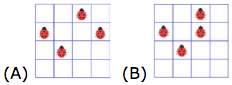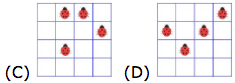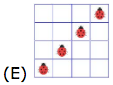1. Part A: Each correct answer is worth 3 points

Which shape cannot be formed using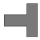and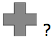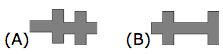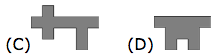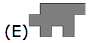2. At least how many 4-ray stars like thisare glued together to make this shape?

3. This pizza was divided into equal slices.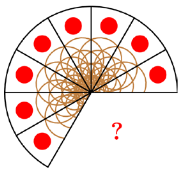How many slices are missing ?

4. How many kangaroos must be moved from one park to the other in order to get the same number of kangaroos in each park?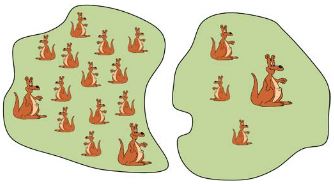5. Which of these ladyb ug s has to fly away so that the rest of them have 20 dots in total?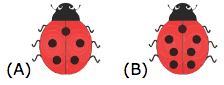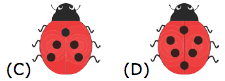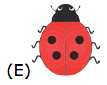6. Emilie builds tower s in the following pattern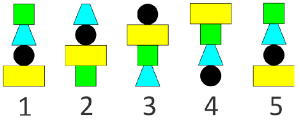Which one will be the tower number 6?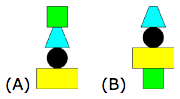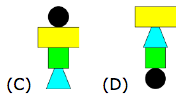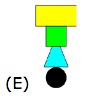7. Part B: Each correct answer is worth 4 points

If ◊ + ◊ = 4 and ∆ + ∆ + ∆ = 9, what is the value of ◊ + ∆ = ?

8. Lisa has 4 pieces, but she only needs 3 for completing her puzzle frame. Which piece will be left over?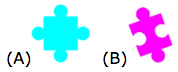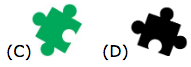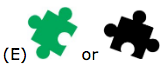9. How many right hands are in this picture?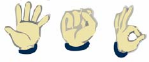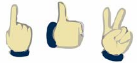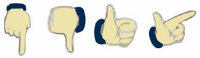10. The dog went to its food following a path. In total it made 3 right turns and 2 left turns. Which path did the dog follow?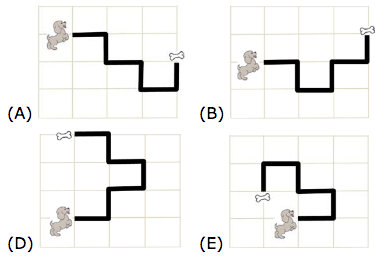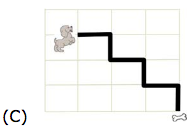11. What number is in the box marked "?" ?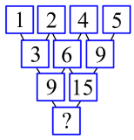12. Charles cut a rope in three equal pieces and then made some equal knots with them. Which figure correctly shows the three pieces with the knots?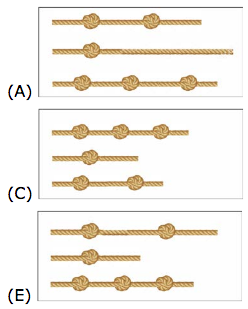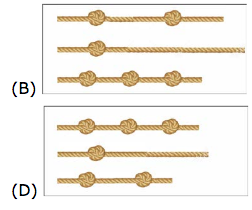13. Part C: Each correct answer is worth 5 points

How many circles and how many squares are covered by the blot in the picture?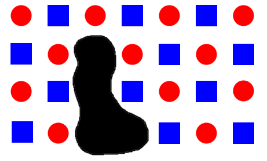14. Diana shoots three arrows at a target.
On her first try, she gets 6 points and the arrows land like this: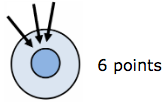On her second try, she gets 8 points and the arrows land like this: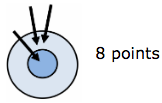On her third try, the arrows land like this: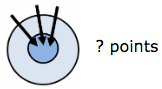How many points wil l she get the third time ?

15. How many different numbers greater than 10 and smaller than 25 with distinct digits can we make by using any two of the digits 2, 0, 1, and 8?

16. Mark had some stick s of length 5 cm and width 1 cm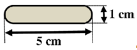. What is the length of the fence?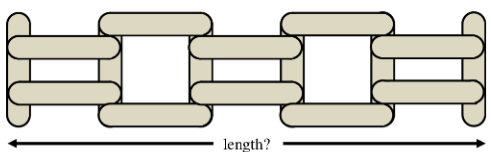17. The road from Anna's house to Mary's house is 16 km long.
The road from Mary's house to John's house is 20 km long .
The road from the crossroad to Mary's house is 9 km long.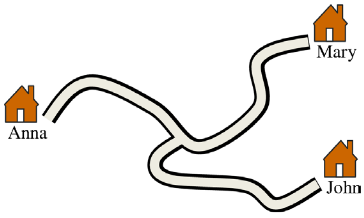How long is the ro ad from Anna’s house to John's house?

18. There are four lady bugs on a 4×4 board. Two are asleep and do not move. The other two ladybugs move one square every minute (up, down, left, or right). Here are pictures of the board for the first four minutes: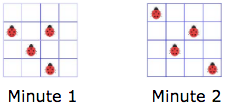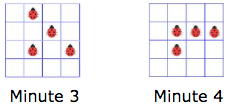Which of these is a picture of the fifth minute (Minute 5) ?